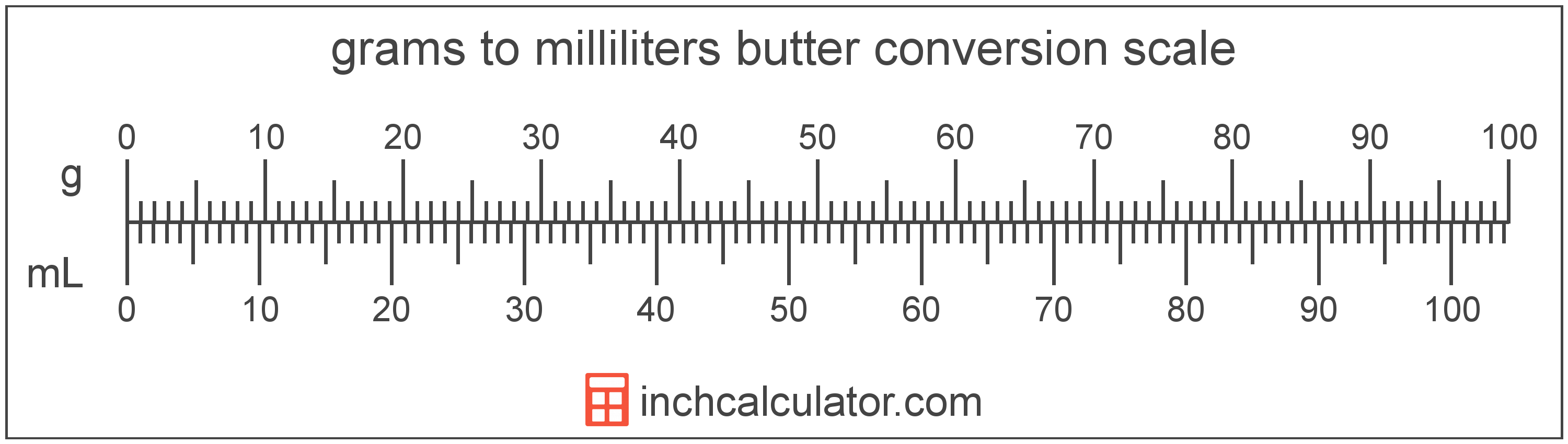# Convert Grams of Butter to Milliliters

Enter the butter in grams below to get the value converted to milliliters.

Results in Milliliters:1 g = 1.043173 mL

Do you want to convert milliliters to grams?

## How to Convert Grams to Milliliters

To convert a measurement in grams to a measurement in milliliters, multiply the butter by the following conversion ratio: 1.043173 milliliters/gram.

Since one gram of butter is equal to 1.043173 milliliters, you can use this simple formula to convert:

milliliters = grams × 1.043173

The butter in milliliters is equal to the butter in grams multiplied by 1.043173.

For example, here's how to convert 5 grams to milliliters using the formula above.
milliliters = (5 g × 1.043173) = 5.215864 mL### How Many Milliliters Are in a Gram of Butter?

There are 1.043173 milliliters in a gram of butter, which is why we use this value in the formula above.

1 g = 1.043173 mL

## What is a Gram?

Butter has a density of 911 grams per liter, and one stick of butter weighs about 113.4 grams.

The gram, or gramme, is an SI unit of weight in the metric system. Grams can be abbreviated as g; for example, 1 gram can be written as 1 g.

## What is a Milliliter?

Butter is sometimes measured by volume in milliliters. One stick of butter contains about 118.3 milliliters.

The milliliter is an SI unit of volume in the metric system. A milliliter is sometimes also referred to as a millilitre. Milliliters can be abbreviated as mL; for example, 1 milliliter can be written as 1 mL.

## Gram to Milliliter Conversion Table

Table showing various gram measurements converted to milliliters.
Grams Milliliters
1 g 1.0432 mL
2 g 2.0863 mL
3 g 3.1295 mL
4 g 4.1727 mL
5 g 5.2159 mL
6 g 6.259 mL
7 g 7.3022 mL
8 g 8.3454 mL
9 g 9.3886 mL
10 g 10.43 mL
11 g 11.47 mL
12 g 12.52 mL
13 g 13.56 mL
14 g 14.6 mL
15 g 15.65 mL
16 g 16.69 mL
17 g 17.73 mL
18 g 18.78 mL
19 g 19.82 mL
20 g 20.86 mL
21 g 21.91 mL
22 g 22.95 mL
23 g 23.99 mL
24 g 25.04 mL
25 g 26.08 mL
26 g 27.12 mL
27 g 28.17 mL
28 g 29.21 mL
29 g 30.25 mL
30 g 31.3 mL
31 g 32.34 mL
32 g 33.38 mL
33 g 34.42 mL
34 g 35.47 mL
35 g 36.51 mL
36 g 37.55 mL
37 g 38.6 mL
38 g 39.64 mL
39 g 40.68 mL
40 g 41.73 mL